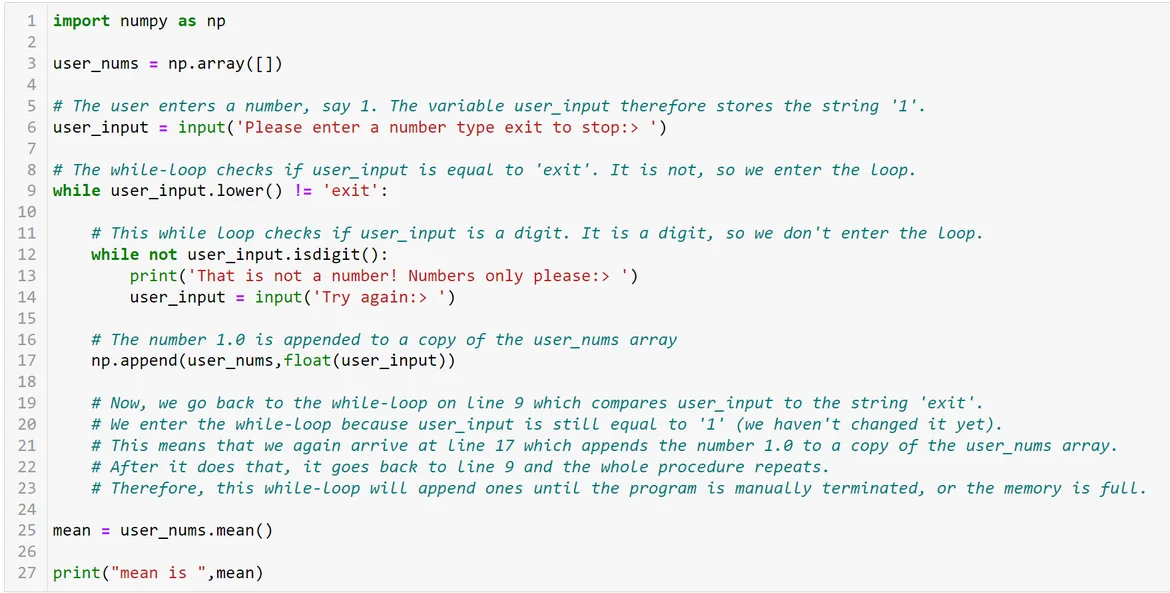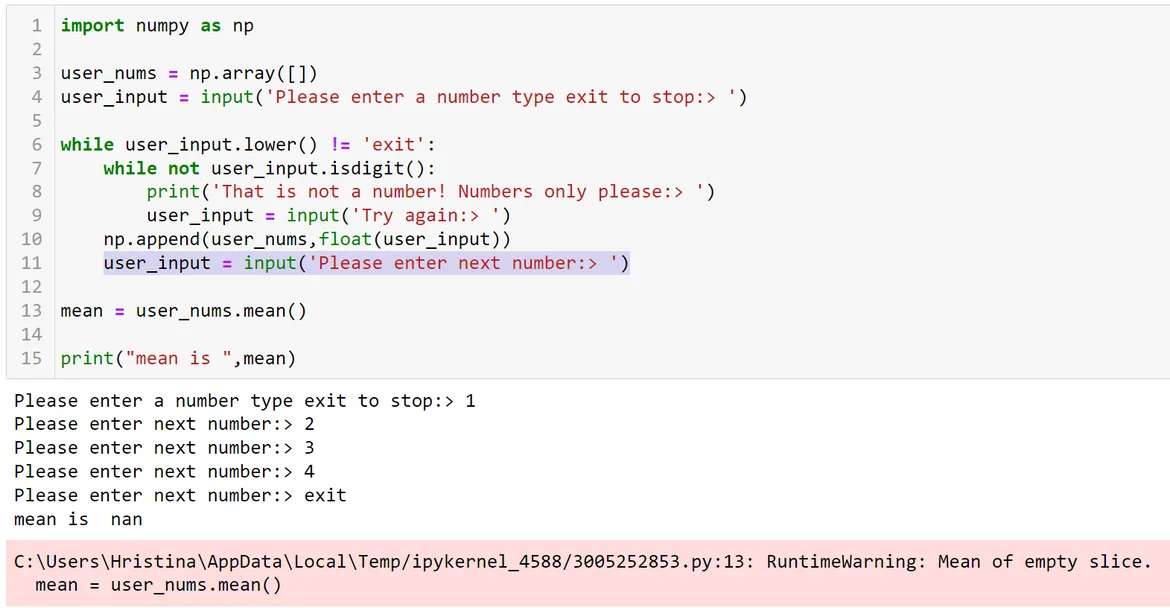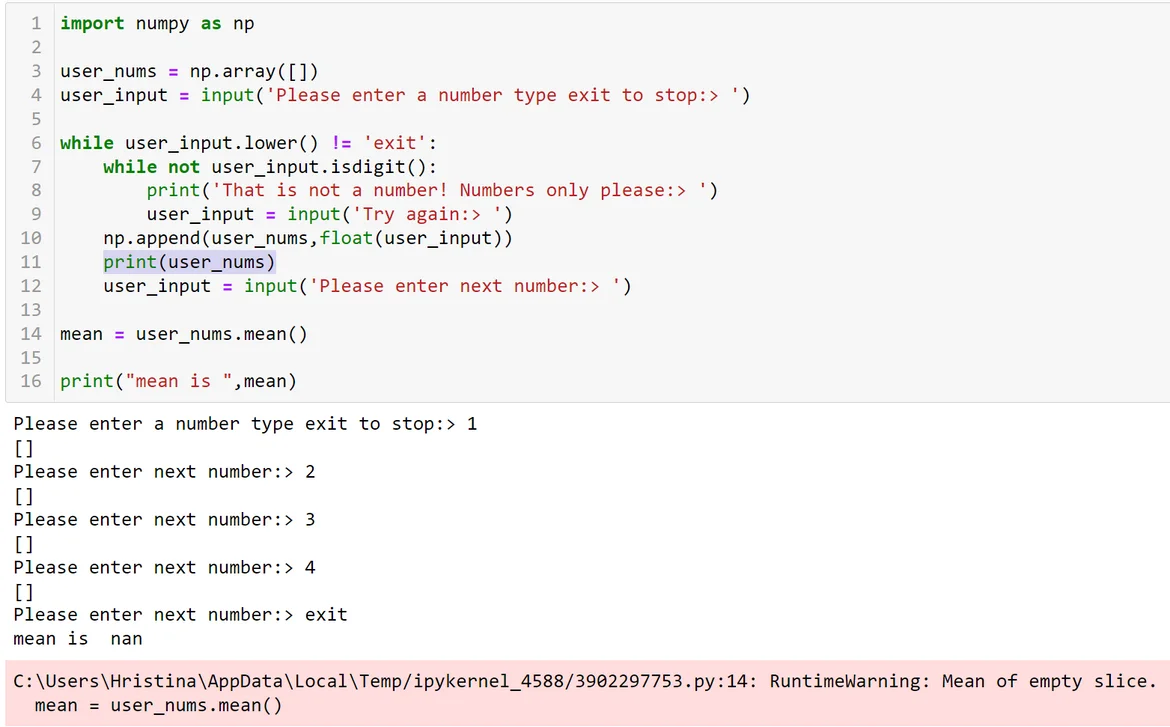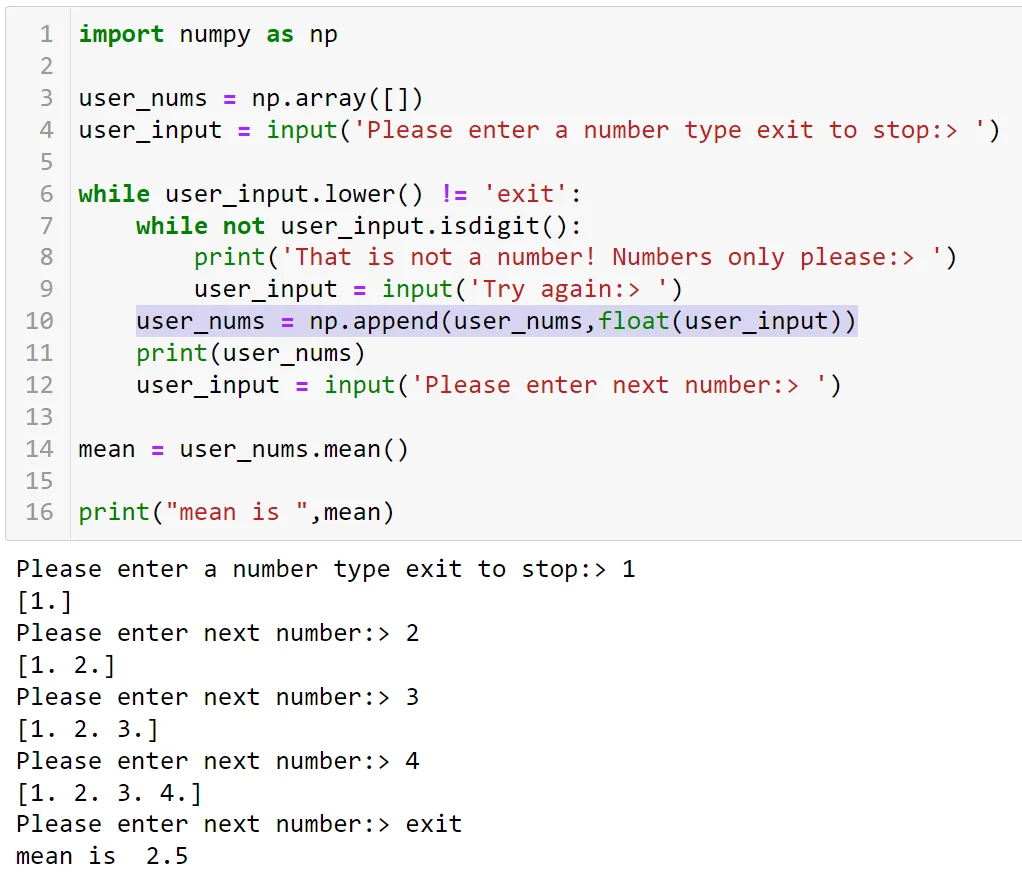14 Jul 2022

Posted on:

14 Jul 2022

1

# Resolved:Section 6 Q5 Python programmer bootcamp

Ask the user to input a sequence of numbers. Then calculate the mean
and print the result.
Here is the solution.

`````` user_input = input('Please enter a number type exit to stop:> ')
numbers = []
while user_input.lower() != 'exit':
while not user_input.isdigit():
print('That is not a number! Numbers only please:> ')
user_input = input('Try again:> ')
numbers.append(int(user_input))
user_input = input('Please enter next number:> ')
total = 0
for number in numbers:
total += number

print(f'Mean is {total/len(numbers)}')
print(sum(numbers)/len(numbers))
``````

Now, my goal is to attempt the question using the following logic and with slight modification to the above code.

• collect the numbers from the user
• store them one by one in a NumPy
• append them one by one in a NumPy array.
• use the np.ndarray.mean to get the mean

## modification to above solution

`````` import numpy as np
user_nums = np.array([])
user_input = input('Please enter a number type exit to stop:> ')
while user_input.lower() != 'exit':
while not user_input.isdigit():
print('That is not a number! Numbers only please:> ')
user_input = input('Try again:> ')
np.append(user_nums,float(user_input))  # append user input to user_nums

mean = user_nums.mean()
print("mean is ",mean)
``````

After inputting the first number, the program takes forever to
continue running leaving me no choice but to force-close the program.
Can you identify my mistakes? Thanks for your kind assistance in

Instructor
Posted on:

14 Jul 2022

1

Hey Carlton,

I have commented your code below:What you need to do in order to avoid entering an infinite loop is to add a line of code asking the user for another number, like so:This time, however, we hit another issue - the mean is `nan`. This suggests that there is nothing stored in the `user_nums` variable. You can convince yourself that if you add a line of code that prints the array every time a number should be appended:In the documentation of the `append()` method, you will find that the method appends the value to a copy of the original array. Therefore, the method creates a copy, stores the value 1.0 there, and leaves the original array unmodified. To populate the original array, you would need to modify the code as follows:Now that works as expected! :)  One should always be extra carful with while-loops.

Hope this helps! Keep up the good work!

Kind regards,
365 Hristina

Posted on:

14 Jul 2022

0

@Hristina. Phenomenally well explained.